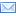Email this page## Doing Fractions with Pattern Blocks

Undergirding the paper-and-pencil manipulations of linear-sequential abstract math symbols is the world of 2-dimensional pictorial representations and 3-dimensional spatial representations with manipulatives. This is an interactive world, and it is at our fingertips for exploring mathematics. The beauty of it is that it allows us to literally SEE and DO the math. This makes math understandable, doable, satisfying and enjoyable. Perhaps for the first time.

Participants will model, in 2 and 3 dimensions, the concepts and skills of equivalent fractions, ordering fractions, finding common denominators, and operations with fractions. We will roll dice and play games, add and subtract fractions without finding common denominators, multiply fractions without changing to improper fractions, and divide fractions without inverting and multiplying, learning different ways to write the operations. We will discover rich new avenues of student feedback and assessment.